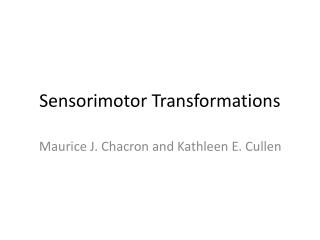DownloadDownload PresentationSensorimotor Transformations

# Sensorimotor Transformations

Download Presentation## Sensorimotor Transformations

- - - - - - - - - - - - - - - - - - - - - - - - - - - E N D - - - - - - - - - - - - - - - - - - - - - - - - - - -
##### Presentation Transcript

1. Sensorimotor Transformations Maurice J. Chacron and Kathleen E. Cullen

2. Outline • Lecture 1: - Introduction to sensorimotor transformations - The case of “linear” sensorimotor transformations: refuge tracking in electric fish - introduction to linear systems identification techniques - Example of sensorimotor transformations: Vestibular processing, the vestibulo-occular reflex (VOR).

3. Outline • Lecture 2: - Nonlinear sensorimotor transformations - Static nonlinearities - Dynamic nonlinearities

4. Lecture 1 Sensorimotor transformation: if we denote the sensory input as a vector S and the motor command as M, a sensorimotor transformation is a mapping from S to M : M =f(S) Where f is typically a nonlinear function

5. Examples of sensorimotor transformations • Vestibulo-occular reflex • Reaching towards a visual target, etc…

6. Example: Refuge tracking in weakly electric fish

7. Refuge tracking

8. Refuge tracking Sensory input Motor output Error

9. Results • Tracking performance is best • when the refuge moves slowly • Tracking performance degrades when • the refuge moves at higher speeds • There is a linear relationship between sensory input and motor output (Cowan and Fortune, 2007)

10. Linear systems identification techniques

11. Linear functions • What is a linear function? • So, a linear system must obey the following definition:

12. Linear functions (continued) • This implies the following: a stimulus at frequency f1 can only cause a response at frequency f1

13. Linear transformations assume output is a convolution of the input with a kernel T(t) with additive noise. We’ll also assume that all terms are zero mean. • Convolution is the most general linear • transformation that can be done to a signal

14. An example of linear coding: • Rate modulated Poisson process time dependent firing rate time

15. Linear Coding: Example: Recording from a P-type Electroreceptor afferent. There is a linear relationship between Input and output Gussin et al. 2007 J. Neurophysiol.

16. Instantaneous input-output transfer function:

17. Fourier decomposition and transfer functions - Fourier Theorem: Any “smooth” signal can be decomposed as a sum of sinewaves • Since we are dealing with linear transformations, • it is sufficient to understand the nature of linear • transformations for a sinewave

18. Linear transformations of a sinewave • Scaling (i.e. multiplying by a non-zero constant) • Shifting in time (i.e. adding a phase)

19. Cross-Correlation Function For stationary processes: In general,

20. Cross-Spectrum • Fourier Transform of the Cross-correlation function • Complex number in general a: real part b: imaginary part

21. Representing the cross-spectrum: : amplitude : phase

22. Transfer functions (Linear Systems Identification) assume output is a convolution of the input with a kernel T(t) with additive noise. We’ll also assume that all terms are zero mean. Transfer function

23. Calculating the transfer function and average over noise realizations multiply by: =0

24. Gain and phase:

25. Sinusoidal stimulationat different frequencies Response Stimulus 20 msec

26. Gain

27. Combining transfer functions input output

28. Where transfer functions fail…

29. Vestibular system Cullen and Sadeghi, 2008

30. Example: vestibular afferents CV=0.044 CV=0.35

31. Regular afferent 120 ` 100 Firing rate (spk/s) 80 60 40 20 Head velocity (deg/s) 0 -20 -40

32. Irregular afferent 160 ` 140 120 100 80 Firing rate (spk/s) 60 40 20 Head velocity (deg/s) 0 -20 -40

33. Signal-to-noise Ratio:

34. Borst and Theunissen, 1999

35. Using transfer functions to characterize and model refuge tracking in weakly electric fish Sensory input Motor output Error

36. Characterizing the sensorimotor transformation 1st order 2nd order

37. Modeling refuge tracking using transfer functions sensory processing sensory input motor processing motor output

38. Modeling refuge tracking using transfer functions sensory processing sensory input motor output Newton